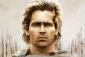# how to find the distance when calculating moment of force

3 posts / 0 new
Mnubi Fatuna Juliahow to find the distance when calculating moment of force

how to find the distance when calculating moment of force

Rate:
fitzmerl duronAlternate questions are highly encouraged. It's too vague....

Rate:
Alexandermoment = force × distance
therefore, distance = moment / force

Rate: